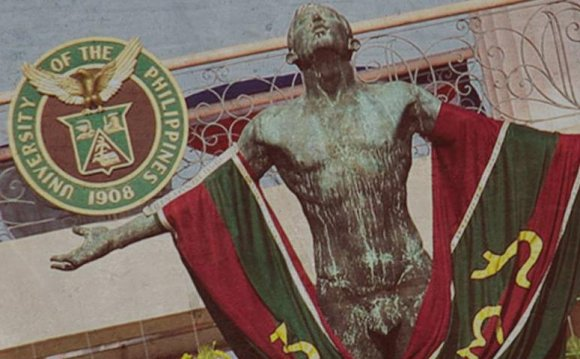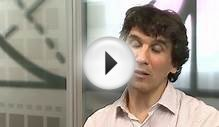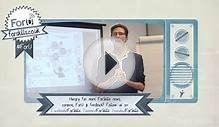# Applied Mathematics major

March 5, 2023In addition to the preliminary and extra-disciplinary requirements described above, the student must earn credit for the following courses, with a grade average of at least 2.0.

• Differential Equations sequence: MAT 414 and MAT 517.
• Numerical Methods course: MAT 581.
• First course in each of the sequences in Table 1.
• Second course in one of the sequences in Table 1.
Table 1: Sequence for B.S. in Applied mathematics
 Sequence First course Second course Linear Transforms MAT 532 or 531 MAT 518 or 682 Analysis MAT 412 MAT 511 or 512 or 513 or 554 Probability and Statistics MAT 521 MAT 525 or 526
• 9 credits of elective mathematics courses numbered 490 or higher. With prior approval of the student's major advisor, mathematically rich 500+ level courses in other departments may be substituted for the mathematics electives.

Distinction in Applied Mathematics is awarded by the Mathematics Department upon completion of a B.S. in Applied Mathematics with a minimum cumulative GPA of 3.4, a minimum GPA of 3.6 in mathematics (MAT) courses at the 300+ level, and either an A or A- in the Senior Seminar or a high-quality Honors Thesis. See the Mathematics Department undergraduate advisor for additional requirements.

Source: math.syr.edu
##### INTERESTING VIDEOPhD in Applied MathematicsPrimary Basic Math Reasoning Skills : Applied Mathematics